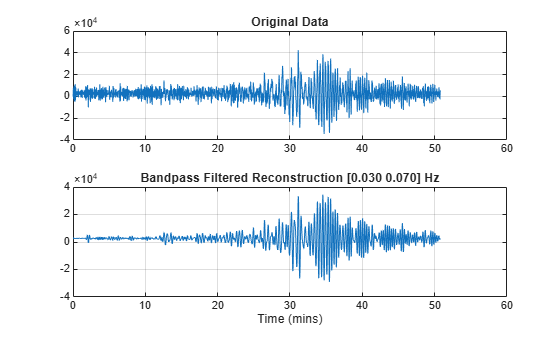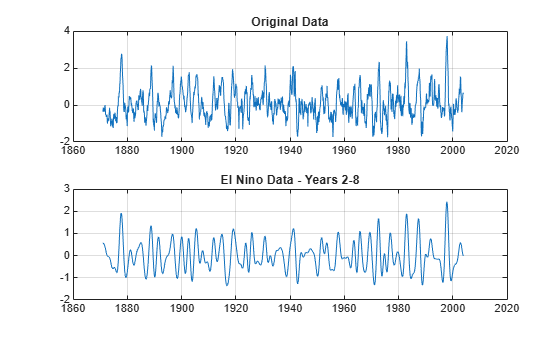Documentation

## Frequency- and Time-Localized Reconstruction from the Continuous Wavelet Transform

Reconstruct a frequency-localized approximation of Kobe earthquake data. Extract information from the CWT for frequencies in the range of [0.030, 0.070] Hz.

`load kobe`

Obtain the CWT of the data.

`[wt,f] = cwt(kobe,1);`

Reconstruct the earthquake data, adding the signal mean back into the transformed data.

`xrec = icwt(wt,f,[0.030 0.070],'SignalMean',mean(kobe));`

Plot and compare the original data and the data for frequencies in the range of [0.030, 0.070] Hz.

```subplot(2,1,1) plot(kobe) grid on title('Original Data') subplot(2,1,2) plot(xrec) grid on title('Bandpass Filtered Reconstruction [0.030 0.070] Hz')```You can also use time periods, instead of frequency, with the CWT. Load the El Nino data and obtain its CWT, specifying the time period in years.

```load ninoairdata [cfs,period] = cwt(nino,years(1/12));```

Obtain the inverse CWT for years 2 through 8.

`xrec = icwt(cfs,period,[years(2) years(8)]);`

Plot the CWT of the reconstructed data. Note the absence of energy outside the band of periods from 2 to 8 years.

```figure cwt(xrec,years(1/12))```Compare the original data with the reconstructed data for years 2 through 8.

```figure subplot(2,1,1) plot(nino) grid on title('Original Data') subplot(2,1,2) plot(xrec) grid on title('El Nino Data - Years 2-8')```##### SupportGet trial now